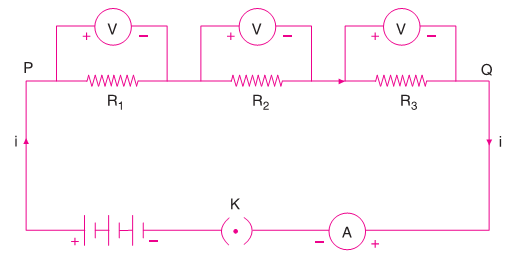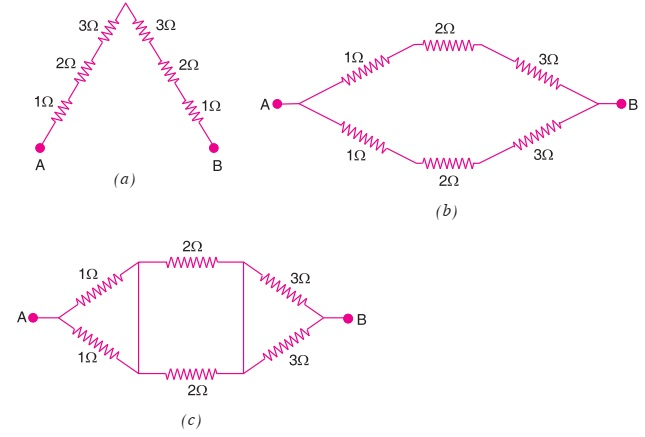# Combination of Resistors

In an electric circuit, resistors can be connected in two different ways: (i) Series Combination: two or more resistors are combined end to end consecutively. (ii) Parallel Combination: two or more resistors can be connected between the same two points.

### Series Combination

When resistors are connected in series with a cell and an ammeter, due to one path the same current i flows through all of them.Let the potential difference between the ends of the resistors R1, R2 and R3 are respectively V1, V2 and V3. By ohm's law potential difference across each resistor

V1 = iR1

V2 = iR2

V3 = iR3

If the potential difference between P and Q be V, then

V = V1 + V2 + V3

V = iR1 + iR2 + iR3

V = i(R1 + R2 + R3)

Let total or equivalent resistance between P and Q is Rs

V = iRs

Rs = R1 + R2 + R3

The equivalent resistance of three resistors connected in series is equal to the sum of their individual resistances.

### Parallel Combination

When resistors connected in parallel with a cell and an ammeter, the potential difference between points P and Q is same across each resistor but the current flows from P to Q is equal to the sum of the separate currents passing through each branch of a given resistance.If i1, i2 and i3 respectively represent the current passing through the branches having the resistors R1, R2, and R3 then the total current i in the main circuit is

i = i1 + i2 + i3

If V is the potential difference across each of the resistors, then according to Ohm’s law

i1 = V/R1

i2 = V/R2

i3 = V/R3

If RP is the equivalent resistance of the resistors connected in parallel having the same potential difference V then

i = V/Rp

V/Rp = V/R1 + V/R2 + V/R3

1/Rp = 1/R1 + 1/R2 + 1/R3

The sum of the reciprocals of the separate resistances is equal to the reciprocal of equivalent or total or resultant resistor Rp.

### Example

Example 1: Find the equivalent resistance of the following combination of resistors.(a) Here, all resistors are connected in series.

R = 1 + 2 + 3 + 3 + 2 + 1 = 12 Ω

(b) Here, we have two series combinations of 3 resistors, each connected in parallel.

R1 = 1 + 2 + 3 = 6 Ω

R2 = 1 + 2 + 3 = 6 Ω

R = (R1 x R2)(R1 + R2)

= 36/12

= 3 Ω

(c) Here, we have 3 parallel combinations of 2 resistors, each connected in series.

R1 = 0.5 Ω

R2 = 1 Ω

R3 = 1.5 Ω

R = R1 + R2 + R3

R = 3 Ω